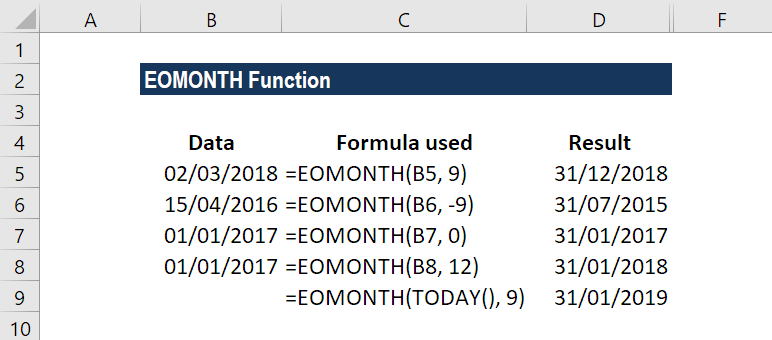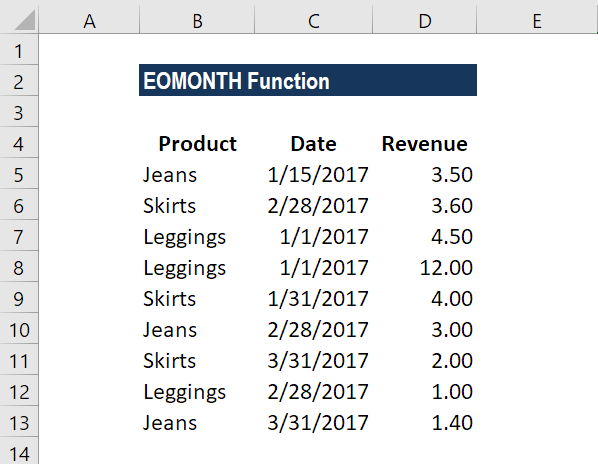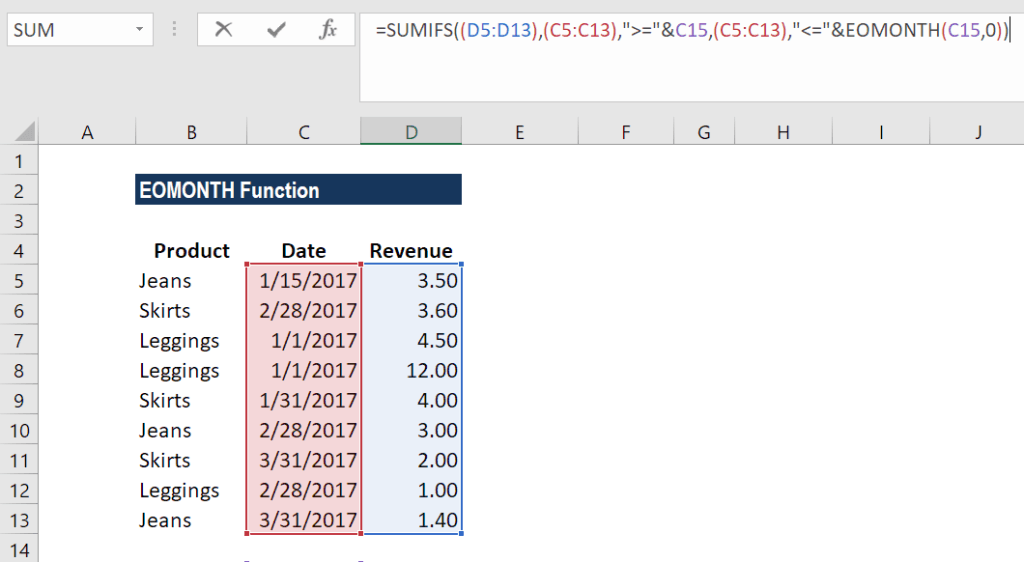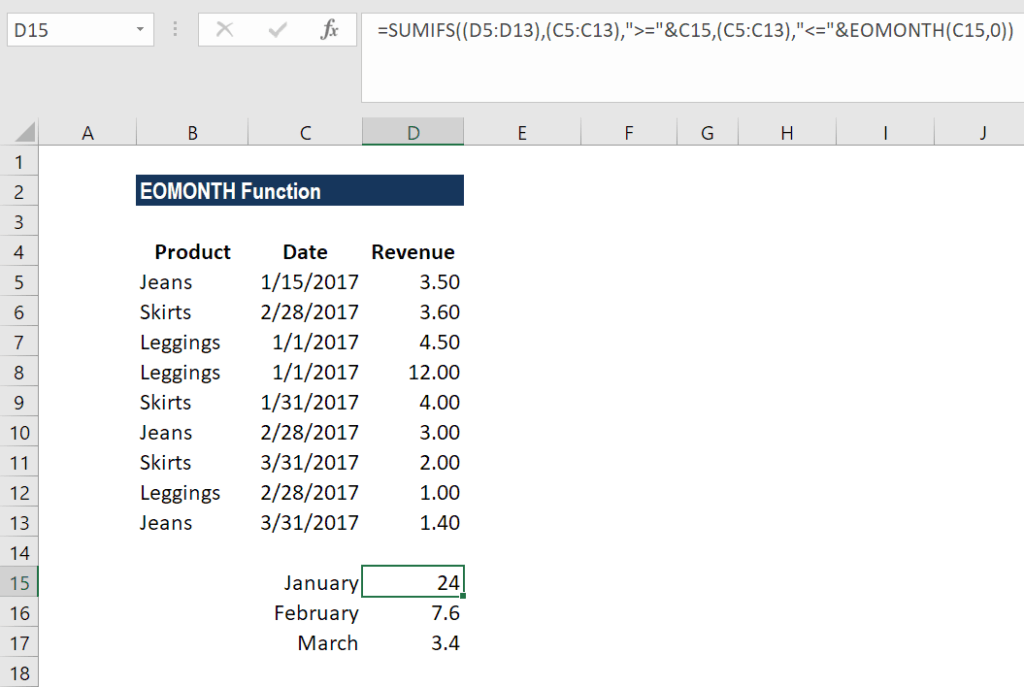# EOMONTH Function

Calculate the last day of the month after adding a specified number of months to a date

## What is the EOMONTH Function?

The EOMONTH Function is categorized under Excel Date/Time functions. The function helps to calculate the last day of the month after adding a specified number of months to a date.

As a financial analyst, the EOMONTH function becomes useful when we are calculating maturity dates for accounts payable or accounts receivable that fall on the last day of the month. It also helps in calculating due dates that fall on the last day of the month. In financial analysis, we often analyze revenue generated by an organization. The function helps us do that in some cases.

### Formula

=EOMONTH(start_date, months)

The EOMONTH function uses the following arguments:

1. Start_date (required argument) – This is the initial date. We need to enter dates in date format either by using the DATE function or as results of other formulas or functions. For example, use DATE(2017,5,13) for May 13, 2017. This function will return errors if dates are entered as text.
2. Months (required argument) – This is the number of months before or after start_date. A positive value for months yields a future date; a negative value produces a past date.

### How to use the EOMONTH Function in Excel?

To understand the uses of this function, let’s consider a few examples:

#### Example 1

Let’s see what results we get when we provide the following data:In Row 2, the function added 9 to return the last day of December (9+3). In Row 3, the function subtracted 9 to return July 31, 2015. In Row 4, the function just returned January 31, 2017. In Row 5, the function added 12 months and returned the last day in January.

In Row 9, we used the function TODAY so EOMONTH took the date as of today that is November 18, 2017, and added 9 months to it to return August 31, 2018.

Remember that the EOMONTH function will return a serial date value. A serial date is how Excel stores dates internally and it represents the number of days since January 1, 1900.

#### Example 2 – Using EOMONTH with SUMIF

Assume we are given the sales of different products in the following format:Now we wish to find out the total revenue that was achieved for the month of January, February, and March. The formula we will use is:

SUMIFS((D5:D13),(C5:C13),”>=”&C15,(C5:C13),”<=”&EOMONTH(C15,0))The result we get is:The SUMIFS formula added up all sales that occurred in January to give 24 (3.5+4.5+12+4) as the result for January. Similarly, we got the results for February and March.

What happened in the formula above is that SUMIFS function totaled up the sales for the range given using two criteria:

1. One was to match dates greater than or equal to the first day of the month.
2. Second, to match dates less than or equal to the last day of the month.

The formula worked in this way:

=SUMIFS(revenue, (C5:C13),”>=”&DATE(2017,1,1), (C5:C13),”<=”&DATE(2017,1,31))

Remember in column E, we must first type 1/1/2017. Then, using a custom format, change it to a custom date format (“mmmm”) to display the month names. If we don’t do it, the formula will not work properly.

Using concatenation with an ampersand (&) is necessary when building criteria that use a logical operator with a numeric value. Hence, we added it to the formula.

### Things to remember about the EOMONTH Function

1. #NUM! error – Occurs if either:
• The supplied start_date is not a valid Excel date; or
• The supplied start_date plus the value of the ‘months’ argument is not a valid Excel date.
2. #VALUE error – Occurs if any of the supplied arguments are non-numeric.
3. If we provide a decimal value for months, the EOMONTH function will only add the integer portion to start_date.

Thanks for reading CFI’s guide to important Excel functions! By taking the time to learn and master these functions, you’ll significantly speed up your financial analysis. To learn more, check out these additional CFI resources:

• Excel Functions for Finance